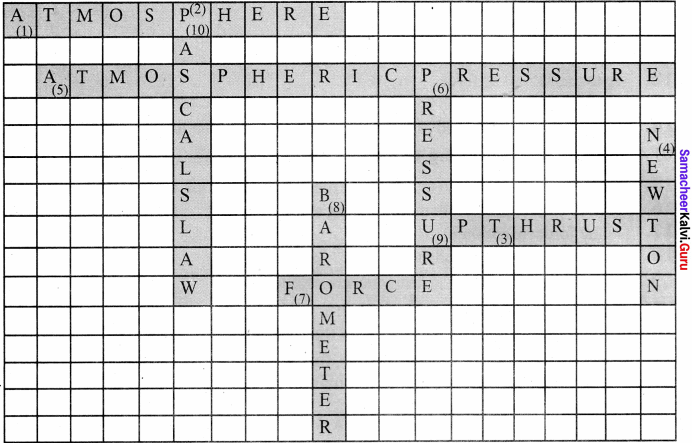## Tamilnadu Samacheer Kalvi 8th Science Solutions Term 1 Chapter 2 Forces and Pressure

### Samacheer Kalvi 8th Science Forces and Pressure Text Book Exercises

Define One Atmosphere Class 8 Question 1.
If we apply a force against the direction of motion of a body, then the body will –
(a) Stop moving
(b) Move with an increased speed
(c) Move with a decreased speed
(d) Move in a different direction
(a) stop moving

Force And Pressure Class 8 Book Back Answers Question 2.
Pressure exerted by a liquid is increased by –
(a) The density of the liquid
(b) The height of the liquid column
(c) Both (a) & (b)
(d) None of the above
(c) Both (a) & (b)

Force And Pressure Class 8 Samacheer Question 3.
Unit of pressure is –
(a) Pascal
(b) N m-2
(c) Poise
(d) Both (a) & (b)
(d) Both (a) & (b)]

Force And Pressure Class 8 Samacheer Kalvi Question 4.
The value of the atmospheric pressure at sea level is –
(a) 76 cm of mercury column
(b) 760 cm of mercury column
(c) 176 cm of mercury column
(d) 7.6 cm of mercury column
(a) 76 cm of mercury column

Samacheer Kalvi Guru 8th Science Question 5.
Pascal’s law is used in –
(a) Hydraulic lift
(b) Brake system
(c) Pressing heavy bundles
(d) All the above
(d) All the above

Define One Atmosphere Answer Question 6.
Which of the following liquids has more viscosity?
(a) Grease
(b) Water
(c) Coconut oil
(d) Ghee
(a) Grease

8th Standard Science Force And Pressure Question 7.
The unit of viscosity is –
(a) N m2
(b) Poise
(c) kg m s-1
(d) No unit
(b) poise

II. Fill in the blanks

1. The pressure of a liquid column …………….. with the depth of the column.
2. Hydraulic lift works under the principle of ………………
3. The property of …………… of a liquid surface enables the water droplets to move upward in plants. [Ans. surface tension]
4. A simple barometer was first constructed by ……………..

1. Increases
2. Pascal’s Law
3. Surface tension
4. Torricelli

III. State whether the following statements are true or false

Samacheer Kalvi Question 1.
Force acting on a given area is called pressure.
True

Define Force Class 8 Question 2.
A moving body comes to rest due to friction alone.
True

Force And Pressure For Class 8 Question 3.
A body will sink if the weight of the body is greater than the buoyant force.
True

Question 4.
One atmosphere is equivalent to 1,00,000 newton force acting on one square metre.
True

Question 5.
Rolling friction is slightly greater than the sliding friction.
False
Correct statement:
Rolling friction is slightly lesser than the sliding friction.

Question 6.
Friction is the only reason for the loss of energy.
True

Question 7.
Liquid pressure decreases with the decrease of depth.
True

Question 8.
Using barometers, one can measure the height of a building.
False
Correct statement:
Using barometers, one can measure the atmospheric pressure.

Question 9.
Surface tension causes the spherical nature of a water drop.
True

Question 10.
Viscosity depends on the pressure of a liquid.
True

IV. Arrange the following in the increasing order

Question 1.
Rolling friction, static friction, sliding friction
Static friction, Rolling friction, Sliding friction.

Question 2.
Let a marble roll on the following surfaces. Arrange the choice of the material such that a marble moving over it covers a greater distance.
Cotton cloth, glass plate, paper, card board, silver plate
Glass plate, silver plate, paper, cotton cloth, card board.

V. Match the following

Question 1.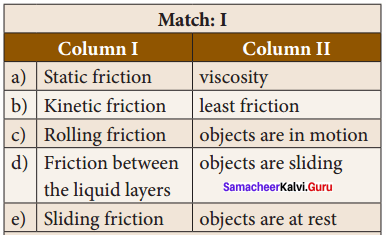i. e
ii. c
iii. b
iv. a
v. d

Question 2.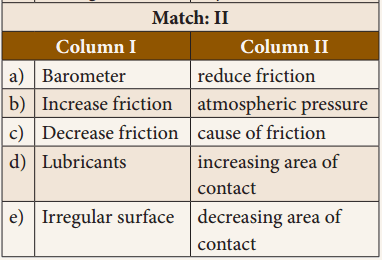i. b
ii. d
iii. e
iv. a
v. c

VI. Analogy

Question 1.
Knot in a thread : ______ friction :: ball bearing : friction ______
Rolling, Static.

Question 2.
Downward force : weight :: Upward force offered by liquid : ______
Buoyant force.

VII. Problems

Question 1.
A stone weighs 500 N. Calculate the pressure exerted by it if it makes a contact with a surface of area 25 cm2.
Given : Weight of a stone F = 500 No
Area A = 25 cm2 = 25 x 10-4 m2
To find : Pressure P = ?
Formula :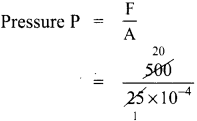Solution:
Pressure P = 20 x 104N/m2 (or) 20 x 104 Pa

Question 2.
In a hydraulic lift, the surface area of the input piston is 10 cm2. The surface area of the output piston is 3000 cm2. A 100 N force applied to the input piston raises the output piston. Calculate the force required to raise the output piston.
Solution:
Pressure input on piston,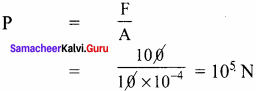According to Pascal’s law
P =$$\frac { F }{ A}$$
P = $$\frac { F}{ A }$$
105 = $$\frac { F }{ 3000 x 10-4}$$ = $$\frac { F x 10-4}{ 3000 }$$
l0-4 x F = 105 x 30OO
F = 3000×101
= 3 x 104 N

VIII. Assertion & Reason

Mark the correct choice as :
(a) If both assertion and reason are true and the reason is the correct explanation of the assertion.
(b) If both assertion and reason are true, but the reason is not the correct explanation of the assertion.
(c) If the assertion is true, but the reason is false.
(d) If the assertion is false, but the reason is true.

Question 1.
Assertion : Sharp knives are used to cut the vegetables.
Reason : Sharp edges exert more pressure.
(a) Both assertion and reason are true and the reason is the correct explanation of the assertion

Question 2.
Assertion : Broad straps are used in bags.
Reason : Broad straps last for long life.
(b) Both assertion and reason are true, but the reason is not the correct explanation of the assertion
Correct explanation:
The weight of the bags falls on larger area of shoulder. So lesser pressure is produced.

Question 3.
Assertion : Water strider slides easily on the surface of water.
Reason : Water strider experiences less buoyant force.
(b) If both assertion and reason are true, but reason is not the correct explanation of assertion
Correct explanation :
It is due to the surface tension of water.

IX. Answer the following in one or two sentences (LOT)

Question 1.
Give two examples to verify that a force changes the shape of a body.
Force can change the static condition of a body.

1. If you squeeze a sponge, its shape changes.
2. If you pull a rubber band, it becomes longer.

Question 2.
Give two examples to verify that a force tends to change the static condition of a body.
Force can change the static condition of a body.

1. A rest rubber ball begins to move, when a force applied on it.
2. Player applies a force on the stationary football while taking a penalty kick is football match. The force applied by player makes the football move towards the goal.

Question 3.
Taking out paste from a tooth paste tube is an example to highlight which physical property?
Pascal’s law.

Question 4.
What do you feel when you touch a nail immediately after it is hammered into a wooden plank? Why?
The nail becomes hot due to friction. Friction changes kinetic energy to heat.

Question 5.
How does the friction arise between the surfaces of two bodies in relative motion?
The force of friction is arised by the interlocking of the irregularities of the two surfaces.

Question 6.
Name two instruments, which help to measure the pressure of a fluid.

1. Manometer
2. Pressure gauge.

Question 7.
Define one atmosphere.
The pressure exerted by this mercury column is considered as the pressure of magnitude ‘one atmosphere’ (1 atm).

Question 8.
Why are heavy bags provided with broad straps?
Broader straps are provided on a back – pack for giving less pressure on the shoulders by providing a larger area of contact with the shoulder.

Question 9.
How does surface tension help a plant?
Water molecules rise up due to surface tension. Xylem tissues are very narrow vessels present in plants. Water molecules are absorbed by the roots and these vessels help the water to rise upward due to “capillarity action” which is caused by the surface tension of water.

Question 10.
Which has greater viscosity, oil or honey? Why?
Honey has greater viscosity.
Reason : Thicker liquids are more viscous than thinner liquids. As honey has greater viscosity, more frictional force will be acting on it.

X. Answer the following questions with a few sentences (MOT)

Question 1.
Define friction. Give two examples of the utility of friction in day to day life.
Friction : Friction is a force that shows down moving objects or prevents stationary objects from moving.
Examples of the utility of friction in day to day life.

• Cars and buses are able to move safely on the road because of friction between the treaded tyres and the surface of the road.
• We are able to write on paper only with the help of friction between the pencil or pen and paper.

Question 2.
Write down three ways of minimising friction.
1. By using lubricants:
These are applied to surfaces to reduce the friction between the surfaces. Example Oil, wax, grease and castor oil.

2. With the help of polishing the surface:
We sprinkle fine powder on the carrom board and then we polish its surface to make smooth so that the striker slides easily on the surface.

3. By using ball bearing:
We use leadshots in bearing of a cycle hub because rolling friction is smaller than sliding friction.

Question 3.
How do sailors protect their ship during a heavy storm?
During a heavy storm, sailors pour soap powder or oil into the sea near their ship to decrease the surface tension of sea water. This process reduces the impact of the violent water current against the wall of ship.

Question 4.
Write down three applications of Pascal’s law.

1. In an automobile service station, the vehicles are lifted upward using the hydraulic lift, which works as per Pascal’s law.
2. The automobile brake system works according to Pascal’s law.
3. The hydraulic press is used to make the compressed bundles of cotton or cloth so as to occupy less space.

Question 5.
Why is a ball bearing used in a cycle hub?
The rolling friction is smaller than sliding friction, sliding is replaced by rolling with the usage of ball bearings. So lead shots are used in the bearing of a cycle hub.

XI. Answer the following questions in detail

Question 1.
“Friction is a necessary evil”- explain.
Friction is a necessity in most of our day to day activities. It is desirable in most situations of our daily life.

1. We can hold any object in our hand due to friction.
2. We can walk on the road because of friction. The footwear and the ground help us to walk without slipping.
3. Writing easily with a pen on paper is due to friction.
4. Automobiles can move safely due to friction between the tyres and the road. Brakes can be applied due to frictional resistance on brake shoes.
5. We are able to light a matchstick, sew clothes, tie a knot or fix a nail in the wall because of friction.

Though it is giving a negative effect, in most of our day to day life friction helps us to make our life easy. So, it is called as “necessary evil”.

1. Friction wears out the surfaces rubbing with each other, like screws and gears in machines or soles of shoes.
2. To overcome the friction an excess amount of effort has to be given to operate a machine. This leads to wastage of energy.

Question 2.
Give the different types of friction and explain each with an example.
Friction can be classified into two basic types:

1. Static friction
2. Kinetic friction.

1. Static friction : The friction experienced by the bodies, which are at rest is called static friction. (E.g : All the objects rigidly placed to be at rest on the Earth, a knot in a thread.

2. Kinetic friction : Friction existing during the motion of bodies is called kinetic friction.

Further, kinetic friction can be classified into two:

• Sliding friction
• Rolling friction.

1. Sliding friction : When a body slides over the surface of another body, the friction acting between the surfaces in contact is called sliding friction.

2. Rolling friction : When a body rolls over another surface, the friction acting between the surfaces in contact is called rolling friction. Rolling friction is less than sliding friction. That is why wheels are provided in vehicles, trolleys, suitcases etc.

Question 3.
Describe an experiment to prove that friction depends on the nature of a surface.
To understand about the frictional force between the layers of liquid in motion.
Materials required : Different kinds of liquid (coconut oil, honey, water, ghee), glass plates – 4 nos.
Procedure :

• Take a small quantity of different kinds of liquid like coconut oil, honey, water and ghee etc., in a cup.
• Place one drop of each liquid on a separate glass plate.
• Next, gently raise one end of the glass plate, one by one, so as to allow the liquid to slide down the smooth surface of the plate.
• Observe the speed of each liquid.

Observation:
Each liquid moves with a different speed. Water flows faster than other liquids. Coconut oil flows with a moderate speed. Ghee flows very slowly.

Inference:
Between the layers of each liquid, in motion, there is a frictional force parallel to the layers of the liquid. This frictional force opposes the motion of the liquid layers while they are in motion.

Question 4.
Explain how friction can be minimised.
1. Using lubricants:

• A substance, which reduces the frictional force, is called a lubricant. Example: Grease, coconut oil, graphite, castor oil, etc.
• The lubricants fill up the gaps in the irregular surfaces between the bodies in contact. This provides a smooth layer thus preventing a direct contact between their rough surfaces.

2. Using ball bearing:
Since, the rolling friction is smaller than sliding friction, sliding is replaced by rolling with the usage of ball bearings. We can see lead shots in the bearing of a cycle hub.

Question 5.
Describe an experiment to prove that the pressure in a liquid increases with depth.
Take a plastic bottle. Punch three holes on its side in the same direction, but at different heights. Now pour some water into it and let it flow through the holes. Observe the flow of water.

Inference:
The water comes out from all the holes with a different force and falls on the table at points that are at variable distances from the bottle. Water from the lowest hole comes out with the greatest force and falls at a point that is at the maximum distance from the bottle. Water from the topmost hole comes out with the least force and falls at the point that is at the minimum distance from the bottle.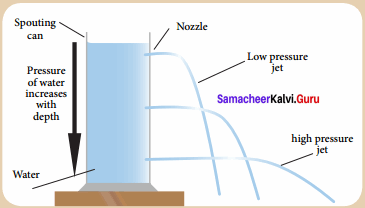Reason:
This activity confirms that the pressure in a liquid varies with the depth of the point of observation in it.

XII. Hot Corner

Question 1.
Why is it not advisable to take a fountain pen while travelling in an aeroplane?
Fountain pens are built in such a way that the pressure inside them balances the atmospheric pressure at sea level. Since atmospheric pressure decreases with an increase in height above sea level, the pressure inside the pen turns out to be much greater than the air pressure in an aeroplane and the pen starts leaking.

Question 2.
Is there any possibility of making a special device to measure the magnitude of friction directly?
Yes. Tribometer is a special device to measure the magnitude of friction directly.

Question 3.
Vidhya posts a question: Mercury is costly. So, instead of mercury can we use water as a barometric liquid? Answer to Vidhya and explain, the difficulty of constructing a water barometer.

1. Mercury is commonly used in barometers because of its high density means the height of the column can be a reasonable size to measure atmospheric pressure.
2. A barometer using water, for instance, would need to be 13.6 times taller than a mercury barometer to obtain the same pressure difference.
3. This is because mercury is 13.6 times more dense than water.

Question 4.
A bubble rises from the bottom of a pond to its surface by increasing its radius by 3 times its value when it was at the bottom. Calculate the depth of the pond. (Hint: Pressure depends on the depth of the pond. Volume is inversely related to pressure.)[Science Olympiad]
Solution:
From Boyle’s law P x $$\frac { 1 }{ v}$$ (Pressure is inversely proportional to volume)
Initial pressure P1 = Atmospheric pressure + Pressure of liquid column
∴ p1 = hpg + Hpg
h = depth of the pond
H = 10 m of water
V1 $$\frac { 4 }{ 3}$$ πr3
P2 = Hpg
V2 = $$\frac { 4 }{ 3}$$ π(3r)3 = $$\frac { 4 }{ 3}$$ x 27 r3 x π
Hence by substituting these values,
P1 V1 = P2 V2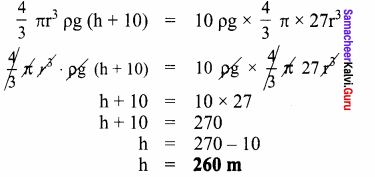### Samacheer Kalvi 8th Science Solutions Forces and Pressure Additional Questions

Question 1.
The SI unit of pressure is ……………..
(a) $$\frac { kg }{ { m }^{ 3 } }$$
(b) $$\frac { kg }{ { m }^{ 2 } }$$
(c) Pascal
(d) Newton
(c) Pascal

Question 2.
The wear and tear in the machine part is due to …………….
(a) Electrostatic force
(b) Frictional force
(c) Muscular force
(d) Gravitational force
(b) frictional force

Question 3.
Which of the following increases friction?
(a) Lubricant
(c) Streamlining
(d) Polishing

Question 4.
The total force exerted by a body normal to the surface is called …………….
(a) Pressure
(b) Thrust
(c) force of gravity
(d) None of these
(b) Thrust

Question 5.
The atmospheric pressure on the surface of the earth is about ………………
(a) 10<sup-5 Nm-2
(b) 104Nm-2
(c) 105 Nm-2
(d) 103Nm-2
(c) 105 Nm-2

Question 6.
The SI unit of force is ……………
(a) Dyne
(b) Newton
(c) Pascal
(d) Newton second
(b) Newton

II. Fill in the Blanks

1. If the same force is made to act on a larger area, the pressure …………….
2. At the given depth, a liquid exerts 1 pressure in all directions.
3. The pressure exerted by the air around us is called …………… pressure.
4. At higher altitudes, atmospheric pressure is …………….
5. Friction depends on the ……………… of two surfaces in contact.

1. Decreases
2. Equal
3. Atmospheric
4. Less
5. Nature

III. True or False – if false give the correct statement:

Question 1.
A push or pull on an object is called force.
True.

Question 2.
Pressure can be increased by decreasing the force.
False
Correct statement:
Pressure can be increased by increasing the force.

Question 3.
All flowing substances such as liquids or gases are called fluids.
True

Question 4.
The pressure exerted by air is called atmospheric pressure.
True

Question 5.
Pressure is directly proportional to the area of contact.
False
Correct statement:
Pressure is inversely proportional to the area of contact.

Question 6.
The pressure in a liquid is the same at all depths.
False
Correct statement:
The pressure in a liquid increases with depth.

IV. Match the following

Question 1.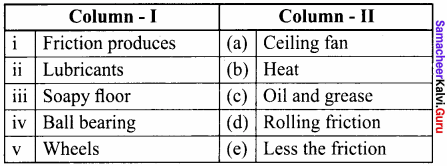i. b,
ii. c
iii. e
iv. a
v. d

Question 2.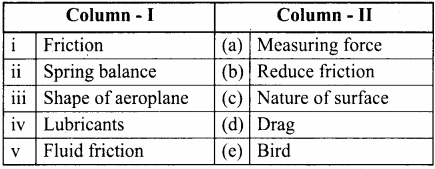i. c
ii. a
iii. e
iv. b
v. d

V. Analogy

Question 1.
Liquid pressure : ______ :: Atmospheric Pressure :______.
Manometer, Barometer.

Question 2.
Broader straps : ______ :: Thin needles : ______.
Lowers pressure, High pressure.

Question 3.
Pascal’s law : ______:: Surface tensin : ______.
Hydraulic brake, Capillary action.

Question 4.
Viscous force : ______ :: Buoyant force : ______.
Viscosity, Buoyancy.

Question 5.
Objects placed at rest on earth : ______ :: Bodies slide over the surface on other body : ______.
Static friction, Sliding friction.

VI. Assertion and Reason

Mark the correct choice as :
(a) If both assertion and reason are true and the reason is the correct explanation of the assertion.
(b) If both assertion and reason are true, but the reason is not the correct explanation of the assertion.
(c) If the assertion is true, but the reason is false.
(d) If the assertion is false, but the reason is true.

Question 1.
Assertion : Force is defined as a push or pull acting on a body.
Reason : CGS unit of force is newton.
(c) The assertion is true, but the reason is false

Question 2.
Assertion : Friction always opposes the motion.
Reason : Whenever one surface moves or tries to move over another surface, the force of friction starts acting on the surfaces.
(d) The assertion is false, but the reason is true

Question 3.
Assertion : The pressure at the bottom of the sea is lesser than that near the surface.
Reason : The pressure exerted by a liquid depends upon the depth of the liquid and density of the liquid.
(d) The assertion is false, but the reason is true

Question 4.
Assertion : We can live very happily if friction is not present in nature.
Reason : Aeroplane shape is streamlined to reduce the effort of frictional force.
(d) The assertion is false, but the reason is true

Question 5.
Assertion : There is danger of a vehicle skidding on a wet road.
Reason : The tyres of the vehicle lose their grip on the road due to increase in friction due to presence of water on the road.
(c) The assertion is true, but the reason is false

Question 1.
Write the SI unit of force.
Newton (N).

Question 2.
Write the SI unit of pressure.
Pascal (Pa).

Question 3.
Mention the factors that the effect of a force depend.

1. Magnitude of the force
2. The area over which it acts.

Question 4.
Name the material which is used to reduce friction.
Lubricant.

Question 5.
What is lateral pressure?
Liquid exerts pressure on the walls of the container also. This is called lateral pressure.

Question 6.
What is thrust?
The force acting normally on a surface is called thrust.

Question 7.
Name an instrument used to measure the difference in the liquid pressure.
Barometer.

Question 8.
Mention the two types of forces.

1. Contact force
2. Non – contact force.

Question 9.
State whether the liquids and gases also exerts pressure.
Yes, both the liquids and gases also exerts pressure.

Question 10.
In ‘tug of war’ when two teams pull equally hard, then what happens?
In ‘tug of war’ when two teams pull equally hard, then the rope does not move in any direction.

Question 1.
Define force. Mention its SI unit.
Force is that which changes or tends to change:

1. The state of rest or
2. The state of uniform motion of a body or
3. The direction of a moving body or
4. The shape of a body.

Question 2.
Does the palm apply any force on the ball, when we place our palm in front of a moving ball?
Yes, when we place our palm in front of a moving ball, then the palm apply a force on the moving ball and stop it.

Question 3.
Briefly explain how do we experience force in our daily life.
Since, there are many actions which give us the feeling of force like we hit or catch many objects in our regular routine. Most of the times, we see that the moving ball stops after sometime and it changes the direction of motion when it is hit by a bat also, when we compress a spring, its shape and size changes.

Question 4.
Can you lift or push a book lying on a table without touching it?
No, we cannot lift or push a book lying on a table without touching it, because it is a type of contact forces.

Question 5.
Explain the effect on the pressure when area on which it is applied, decreases.
As, we know that pressure is defined as the force acting on a unit area of a surface, then,
Force
Pressure = $$\frac { Force }{ Area}$$
P = $$\frac { F }{ A}$$
p ∝ $$\frac { 1 }{ A}$$
So, pressure is inversely proportional to the area on which force is applied. Therefore with the decrease in area the pressure increases.

Question 6.
Explain the variation of the pressure exerted by liquid with respect to following factors.

1. Amount of liquid
2. Depth
3. Shape and size of container

The variation of pressure exerted by liquid with respect to the above factors can be given by,

1. Pressure in a liquid increases with the amount of liquid.
2. Pressure in a liquid increases with depth.
3. Pressure of liquid is independent of shape and size of container.

Question 7.
Give two examples to reduce friction.

1. Drops of oil are poured on the hinges of a door to move the door smoothly,
2. Fine powder are sprinkled on the carrom board to reduce friction.

Question 8.
Explain why the cutting instruments are sharpened.
Cutting instruments have very small area of cross – section, which lead to the increase in pressure. So they can easily penetrate the surface (pressure is inversely proportional to area). That’s why cutting instruments are usually very much sharpened.

Question 9.
Cooking in a place located at a higher attitude is difficult. Why?
At a higher altitude, due to the lack of atmospheric pressure the boiling point of a substance reduces. So, the water boils even at 80° C. At this temperature, the thermal energy that is produced is not sufficient enough for baking or cooking. So, cooking is difficult at higher altitude.

Question 10.

1. An upward force is exerted by water on a floating or a partly submerged body. This upward force is called buoyant force. The phenomenon is known as “buoyancy”.
2. This force is not only exerted by liquids, but also by gases. Liquids and gases together are called fluids.
3. This upward force decides whether an object will sink or float. If the weight of the object is less than the upward force, then the object will float. If not, it will sink.

Question 1.
Advantages of friction : Friction is a necessity in most of our day to day activities. It is desirable in most situations of our daily life.

1. We can hold any object in our hand due to friction.
2. We can walk on the road because of friction. The footwear and the ground help us to walk without slipping.
3. Writing easily with a pen on paper is due to friction.
4. Automobiles can move safely due to friction between the tyres and the road. Brakes can be applied due to frictional resistance on brake shoes.
5. We are able to light a matchstick, sew clothes, tie a knot or fix a nail in the wall because of friction. Though it is giving a negative effect, in most of our day to day life friction helps us to make our life easy. So, it is called as “necessary evil”.

1. Friction wears out the surfaces rubbing with each other, like screws and gears in machines or soles of shoes.
2. To overcome the friction an excess amount of effort has to be given to operate a machine. This leads to wastage of energy.
3. Friction produces heat, which causes physical damage to the machines.

Question 2.
Define Pascal’s law. Explain the applications of Pascal’s law in our daily life.
Pascal’s law : The pressure applied at any point of a liquid at rest, in a closed system, will be distributed equally through all regions of the liquid.
Application of Pascal’s law : Some of the following examples highlight their working according to Pascal’s law.

1. In an automobile service station, the vehicles are lifted upward using the hydraulic lift, which works as per Pascal’s law.
2. The automobile brake system works according to Pascal’s law.
3. The hydraulic press is used to make the compressed bundles of cotton or cloth so as to occupy less space.

Question 3.
What is surface tension? Explain the applications of surface tension.
Surface tension is the property of a liquid. The molecules of a liquid experience a force, which contracts the extent of their surface area as much as possible, so as to have the minimum value. Thus, the amount of force acting per unit length, on the surface of a liquid is called surface tension.

Application of surface tension:

1. Water molecules rise up due to surface tension. Xylem tissues are very narrow vessels present in plants. Water molecules are absorbed by the roots and these vessels help the water to rise upward due to “capillarity action” which is caused by the surface tension of water.

2. For a given volume, the surface area of a sphere is the minimum. This is the reason for the liquid drops to acquire a spherical shape.

3. Water strider insect slides on the water surface easily due to the surface tension of water.

4. During a heavy storm, sailors pour soap powder or oil into the sea near their ship to decrease the surface tension of sea water. This process reduces the impact of the violent water current against the all of ship.

Question 4.
Briefly explain the concept of variation of atmospheric pressure.

1. Atmospheric pressure vary from place to place and from time to time due to variation in temperature. Even, the density of air decreases with the increase in temperature.
2. So, as a result, the atmospheric pressure also decreases. The atmospheric pressure at a place is because of the weight of air above it.
3. So, if we go higher above the earth surface, the atmospheric pressure decreases, thus at mountain top the pressure is less. The atmospheric pressure at sea level is considered as standard pressure.

X. Thinking Comer : HOTS

Question 1.
Why dams are made stronger and thicker at the bottom that at the top?
This is because the pressure of the water is much greater deeper down and the damneeds to be thick at the bottom so that is strong enough to withstand this larger pressure.

Question 2.
Why do scuba divers wear a special suit while they go into deep sea levels?
Pressure deep under the sea is so high that if the divers don’t wear specially designed suits, the water pressure will crush their body.

Question 3.
Why are rain drop spherical in nature?

1. Surface tension is responsible for the shape of liquid droplets. Although easily deformed, droplets of water tend to be pulled into a spherical shape by the cohesive force of the surface layer.
2. In the absence of other force including gravity drops of virtually all liquids would be approximately spherical.

Question 4.
A liquid flowing out of a very small opening of a tube or tap comes out in the form of fine drops and not as a continuous stream. Why?
For a given volume, the surface area of a sphere is the minimum. This is the reason for the liquid drops to acquire a spherical shape.

Question 5.
Trees are greenish. They are greenish at the tip too. How does the water rise upward in a tree or plant against the force of gravity?

1. Water can travel to great heights inside plants. Water moves up the xylem through a process called capillary action.
2. During transpiration, water evaporates from holes in the surface of leaves into the air. As water molecules evaporate from plant leaves, they attract the water molecules still in the plant, helping to pull water up through the stems from the roots.

XI. Problems for practice

Question 1.
Determine the pressure when a force of 200 N acts on area

1. 20 m2
2. 8 m2

1. Pressure P = $$\frac { F }{ A}$$
F = 200 N, A = 20 m2
P = $$\frac { 200}{ 20}$$= 10N/m2or 10 Pa

2. A = 8 m2
P = $$\frac { 200}{ 8}$$ = 25 N/m2 or 25 Pa

Question 2.
A force of 20 N acts over an area of 4 cm2 Find the value of pressure? (in Nm-2)
Given:
Pressure = $$\frac { F }{ A}$$
F = 20 N
A = 4 cm2 = 4 x 10-2m
Solution:
P = $$\frac { F }{ A}$$ = $$\frac { 20 }{ 4 x 10-2 }$$
= 5 x 102
= 500 Nm-2 (or) 500 pack

Question 3.
What will be the force required to exert a pressure of 20,000 Pa on an area of 1 cm2.
Given:
P = 20,000 Pa
A = 1 cm2 = 1 x 10-4 m2
Formula:
p = $$\frac { F }{ A}$$
F =P x A
Solution:
= 20,000 x 10-4
= 2 x 10-4 x 10-4
= 2 N.

Question 4.
Calculate the area of a 1500 N object that exerts a pressure of 500 Pa.
Given:
P = 500 Pa
F = 1500N
Formula:
p = $$\frac { F }{ A}$$
A = $$\frac { F }{ P}$$
Solution:
= $$\frac { 1500}{ 500}$$
= 3m2

XII. Cross word puzzle

Across:
1. The mixture of gases that surrounds the Earth or some other celestial body.
3. A force acting normal to a surface.
5. The pressure exerted by air.
7. Something that causes a body to move, change its speed or direction, or distorts its shape.
9. The upward force that fluids exert on all matter.

Down:
2. A unit used to measure pressure.
4. A unit used to measure force.
6. Amount of force applied per unit area.
8. An instrument for measuring atmospheric pressure.
10. The pressure exerted at any point on a enclosed liquid is transmitted equally and undiminished in all directions.

1. Atmosphere
3. Thrust
5. Atmospheric Pressure
7. Force
9. Upthrust

Down:
2. Pascal
4. Newton
6. Pressure
8. Barometer
10. Pascals Law